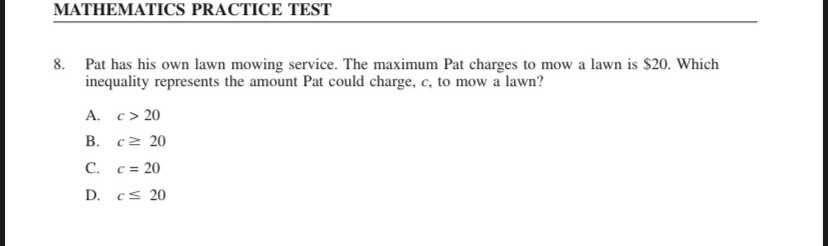### ¿Todavía tienes preguntas de matemáticas?

Pregunte a nuestros tutores expertos
Algebra
Pregunta8. Pat has his own lawn mowing service. The maximum Pat charges to mow a lawn is $$\ 20 .$$ Which inequality represents the amount Pat could charge, $$c ,$$ to mow a lawn? A. $$c > 20$$

B. $$c \geq 20$$

C. $$c = 20$$

D. $$c \leq 20$$